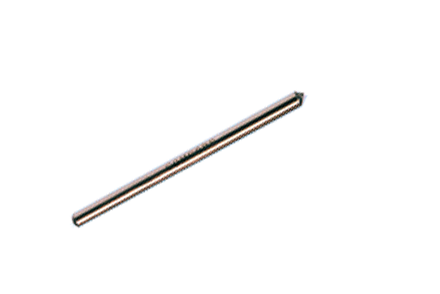### Current Position：Home / Products

 1x2(2x2) Ultra-Low Splitting Ratio TapsProduct Features Product Applications Low Excess Loss High Power Optical Amplifiers Low Insertion Loss Optical Testing Systems High Power Handling Optical Fiber Sensors Stable and High Reliable Fiber Lasers High Power Monitors
 Specifications Tap Port Ratio: 0.1%, 0.01%, 0.001% Parameter Unit Premium A grade Port Configuration 1x2 or 2x2 Bandwidth nm ±20 Insertion Loss for Through Port Max dB 0.1 Insertion Loss for 0.1% Tap Port dB 30±3 30±3 Insertion Loss for 0.01% Tap Port dB 40±4 40±4 Insertion Loss for 0.001% Tap Port dB 50±5 50±5 Excess Loss Typ dB 0.03 Return Loss* Min dB 55 50 Operating Power Max W 5 Operating Temperature °C -40 to +85 Storage Temperature -50 to +85 Fiber Type Single Mode Fiber Package Type mm S6=Ø3x54: for bare fiber S8=Ø3x70: for 0.9mm loose tube M1=9x16x90: for 0.9mm loose tube or 2mm cable or 3mm cable

*Test at central wavelength only. There would be an unused termination port around 20cm for 1x2 version.

 Ordering Information L S T Wavelength 1=1625nm 2=1590nm 3=1570nm 4=1550nm 5=1480nm 6=1475nm 7=1310nm 8=1064nm R=1030nm 9=980nm A=850nm K=830nm L=780nm P=2000nm S=Specify Structure 1=1x2 2=2x2 Splitting Ratio 01=0.1% 02=0.01% 03=0.001% Grade P=Premium A=A grade Package 5=S6 7=S8 D=M1 Fiber Type 1=G652 or Equivalent 5=980-20 6=SM1060 7=SM1060 FLEX 8=980-16 9=SM780C H=SM1950 L=Large mode area fiber Pigtail S=250μm bare fiber M=0.9mm loose tube L=3mm cable R=2mm cable Fiber Length 0=0.5m 1=0.75m 2=1.0m 3=1.5m 4=2.0m S=Specify Connector 0=None 1=FC/PC 2=FC/SPC 3=FC/APC 4=SC/SPC 5=SC/APC 6=ST 7=FC/UPC 8=SC/UPC 9=MU A=LC/PC B=SC/PC C=LC/UPC D=LC/APC

Note:1. Central wavelength can be customized for different applications.
2. All specifications are before connectors and are subject to change without notice.
3. All data are measured at central wavelength at room temperature.